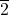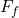## A 1510 kg car is experiencing a 2650 N friction force from the road. What force must be applied to the car in order for the car to move for

Question

A 1510 kg car is experiencing a 2650 N friction force from the road. What force must be applied to the car in order for the car to move forward with a constant velocity of 50.0 km/hr?

in progress 0
2 months 2021-08-02T08:53:52+00:00 1 Answers 1 views 0

The force that must be applied to maintain a constant velocity is equal o the friction force which is 2,650 N

The total force required from rest is (2,650·t + 20,972.)/t N

Explanation:

The given data of the car in motion are;

The mass of the car, m = 1,510 kg

The force of friction from the road,= 2,650 N

When the car is moving at 50.0 km/hr, we have;

v = 50.0 km/hr = 250/9 m/s ≈ 13.88889 m/s

According to Newton’s first Law of motion, a body will continue in a state of rest or in uniform motion along a straight line unless acted on by a force

The force acting on the car in motion = 2,650 N

When the car is moving at a constant velocity, the forces acting on the  car are in equilibrium and the net force is zero

Therefore;

The magnitude of the force that will keep the car moving at a constant velocity = The friction force of the road = 2,650 N

The direction of the force = In the direction of motion of the car

Where the time it takes the car to accelerate from rest to 50.0 km/hr = t seconds, we have;

The force required to move at a constant speed = 1,510 kg × (250/9 m/s – 0)/t s = 20,972./t N

The total force required to move forward with a constant velocity of 50.0 km/hr becomes 20,972./t N + 2,650 N = (2,650·t + 20,972.)/t N

Where;

t = The time it takes the car to reach 50.0 km/hr from rest.